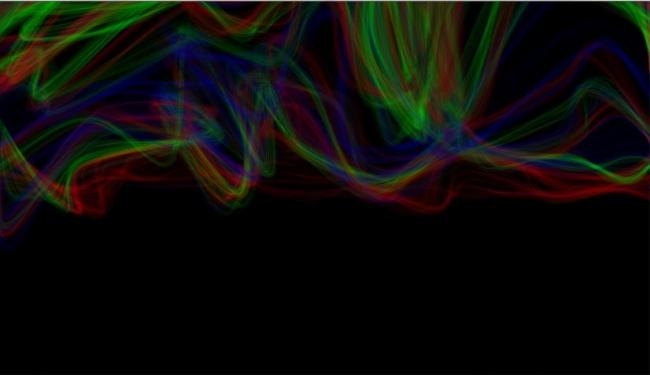#### Howdy, Stranger!

We are about to switch to a new forum software. Until then we have removed the registration on this forum.

# Introduce minim library to my code

edited August 2015

Greeting everyone!

I got done with this sketch a while ago and wished to introduce a minim file. Any further information upon music visualisation and functions and libraries with respect to audio visualisation will help. Thanks :)

``````    ArrayList<PVector>allVectors;

float wanderSpeed = 3.6;
int numSteps = 14;

float lineHue = 2.0;
float lineSat = 0.0;
float lineBright = 1.5;
float colorTrigger = 0.982;

boolean autoplay = true;

void setup()
{
size(1600, 900);
colorMode(HSB, 1.0, 1.0, 1.0, 1.0);
background(0.0);
noFill();

createLine();
randomizeColor();
}

void draw()
{
strokeWeight(1.01);
//randomize color
if (random (0, 1)>colorTrigger)randomizeColor();

stroke(lineHue, lineSat, lineBright, 0.09);
fill(255);

//draw bottom line

beginShape();
for (int i = 0; i <allVectors.size (); i++)
{
PVector vector = allVectors.get(i);
if (i == 0) curveVertex(vector.x, vector.y);
curveVertex(vector.x, vector.y);
if (i == allVectors.size()-1) curveVertex(vector.x,vector.y);

vector.x +=random(-wanderSpeed, wanderSpeed);
vector.y +=random(-wanderSpeed, wanderSpeed);
vector.y -=0.1;

if (i == 0) vector.x = 0;
if (i == allVectors.size()-1) vector.x = width;
}
endShape();

checkAutoplay();
}

void checkAutoplay()
{
if (autoplay)
{
// reset if line has left screen
for (int i = 0; i<allVectors.size (); i++)
{
PVector vector = allVectors.get(i);
if (vector.y < height) return;
}
// reset if boundary check didn't exit functiion
reset();
}
}

void createLine()
{
allVectors = new ArrayList<PVector>();

for (int i=0; i<=numSteps; i++)
{
PVector vector = new PVector(i * width/numSteps, height/2);
}
}

void randomizeColor()
{
lineHue = random(0, 1);
lineSat = random(0, 1);
lineBright = random(0,1);
}

void reset()
{
background(0.0);
createLine();
randomizeColor();
wanderSpeed = random(0.3, 2.0);
numSteps = (int)random(1, 20);
colorTrigger = random(0.99, 0.9999);
}
``````Tagged:

http://code.compartmental.net/tools/minim/

see

http://code.compartmental.net/minim/

``````import ddf.minim.*;

Minim minim;

AudioPlayer groove = null;

void setup() {
size(512, 200, P3D);
minim = new Minim(this);
if (groove==null) {
println ("failed");
exit();
} else {
groove.loop();
} // else
}

void draw() {

background(0);
stroke(255);

if (groove!=null) {
for (int i = 0; i < groove.bufferSize () - 1; i++) {
line(i, 50  + groove.left.get(i)*50, i+1, 50  + groove.left.get(i+1)*50);
line(i, 150 + groove.right.get(i)*50, i+1, 150 + groove.right.get(i+1)*50);
} // for
} // if
}
//
``````
• Hey, Chrisir. Thanks alot! Will look upon it and get back to you. While I just thought isn't minim an existing library by default in the Processing framework?

• ...isn't Minim an existing library by default in the Processing framework?

It certainly is! And both Processing 1 & 2 comes w/ it bundled already!
I simply don't get why @Chrisir would "advise" the latter as an "answer"! @-)

• I am not sure whether the inbuild sound is the same in all functionality as minim (if so the documentation is very shallow) - also I didn't find minim in the list of the lib manager - nvm

• Hey @Chrisir, I did look into that code

``````    import ddf.minim.*;

Minim minim;

AudioPlayer tame = null;

void setup()
{
size(512, 200, P3D);
minim = new Minim(this);
if (tame == null)
{
println("hailed");
exit();
} else
{
tame.loop();
}//else
}

void draw()
{
background(255);
stroke(0);
if (tame != null)
{
for (int i = 0; i<tame.bufferSize () - 1; i++)
{
line(i, 50 + tame.left.get(i)*50, i+1, 50 = tame.left.get(i + 1)*50);
line(i, 150 + tame.right.get(i)*50, i+1, 50 = tame.right.get(i + 1)*50);
}// for
println("value of i", i);
}
}
``````

As it turns out to be, an error is displayed stating "The left - hand side of the assignment must be a variable"

Am I missing something here?

• typos on lines 30 and 31. compare with chrisir's code.

• Error : java.lang.NullPointer exception

when told to compile and run the sketch

:/

• Hey guys, so I'm little puzzled what library I will go ahead to incorporate music visualisation. Would I go ahead with minim or FFT or both?

• FFT is part of minim